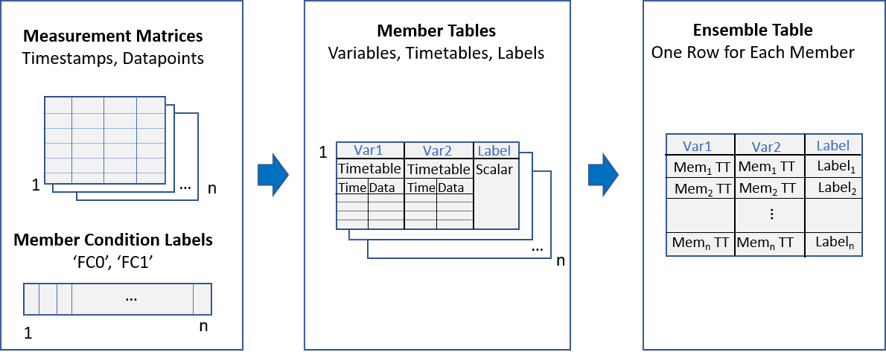Documentation

## Prepare Matrix Data for Diagnostic Feature Designer

This example shows how to prepare matrix data for import into Diagnostic Feature Designer. You step first through conversion of a single-member matrix and its associated fault code into a `table`. Then, you combine multiple tables into a single ensemble table that you can import as a multimember ensemble.

Diagnostic Feature Designer accepts member data that is contained in individual numeric matrices. However, member tables provide more flexibility and ease of use.

• With a table, you can embed your measurements as signal variables, each containing both an independent variable and one or more data variables. With a matrix, you can specify only one independent variable that applies to all the columns of data. The app can more easily interpret table data.

• With a table, you can specify informative variable names. With a matrix, the app uses column numbers to identify data vectors.

• With a table, you can include scalar information for each member, such as condition variables or features. With a matrix, you cannot combine scalar information with signal information. This limitation means that you cannot group data by label in the app, and cannot assess feature effectiveness in separating, say, `healthy` data from `unhealthy` data.

An ensemble table is even easier to use, because it combines all member tables into one dataset. To import an ensemble table, you import just one item, instead of needing to select multiple items.### Load Member Matrices and Fault Codes

Load the member data and fault code data. The member data `dmatall` consists of four sets of timestamped vibration and tachometer measurements taken over an interval of 30 seconds. These member matrices are stacked together in a 3-D matrix. An independent fault code vector `fc` indicates whether each member is from a healthy (`0`) or unhealthy (`1`) system.

Initialize `tv_ensemble table`, which ultimately includes both the time-tagged data and the fault code for each member.

`load tvmatrixmembers dmatall fc`

### Convert a Matrix to a Table

Start by converting a single member matrix to a table that contains timetables for the measurement signals and the member fault code. Extract the first member matrix from `dmatall`.

`memmat = dmatall(:,:,1);`

The first two columns of `memmat` contain the measured vibration signal. The third and fourth contain the measured tacho signal. Each signal consists of an independent variable (time) and a data variable (vibration or tacho measurement). Extract these signals into independent matrices `vibmat` and `tachmat` .

```vibmat = memmat(:,[1 2]); tachmat = memmat(:,[3 4]);```

Convert each signal into a `timetable`. First, separate each signal into its time and data components. Use the function `seconds` to convert the timestamps into duration variables for the timetable. Then input the signal components into `array2timetable` to convert the signals into `timetables` `vibtt` and `tachtt`. Assign the variable name `Data` to the data column. The timetable automatically assigns the name `Time` to the time column.

```vibtime = seconds(vibmat(:,1)); vibdata = vibmat(:,2); tachtime = seconds(tachmat(:,1)); tachdata = tachmat(:,2); vibtt = array2timetable(vibdata,'RowTimes',vibtime,'VariableNames',{'Data'}); tachtt = array2timetable(tachdata,'RowTimes',tachtime,'VariableNames',{'Data'});```

Extract the fault code `faultcode` from the fault code vector `fc`.

`faultcode = fc(1);`

Assemble the member table that contains the two timetables, the fault code scalar, and descriptive variable names.

`memtable = table({vibtt},{tachtt},faultcode,'VariableNames',{'Vibration','Tacho','FaultCode'});`

You now have a member table that you can insert into an ensemble table that contains multiple member tables. Initialize the ensemble table and insert the first member.

```tv_ensemble_table = table(); tv_ensemble_table(1,:) = memtable```
```tv_ensemble_table=1×3 table Vibration Tacho FaultCode ___________________ ___________________ _________ {30001x1 timetable} {30001x1 timetable} 1 ```

### Convert Multiple Member Matrices into Ensemble Table

You can repeat the same processing steps on all member matrices to create the full ensemble table. You can also automate the processing steps for each matrix. To do so, first initialize an ensemble table. Then loop through the member matrices to convert the members to tables and insert them into the ensemble table.

Initialize` tv_ensemble_table`.

`tv_ensemble_table = table();`

Loop through the conversion and insertion sequence

```for idx = 1:size(dmatall,3) vibmat = dmatall(:,[1 2],idx); tachmat = dmatall(:,[3 4],idx); vibtt = array2timetable(vibmat(:,2),'RowTimes',seconds(vibmat(:,1)),'VariableNames',{'Data'}); tachtt = array2timetable(tachmat(:,2),'RowTimes',seconds(tachmat(:,1)),'VariableNames',{'Data'}); tv_member = table({vibtt},{tachtt},fc(idx),'VariableNames',{'Vibration','Tacho','FaultCode'}); tv_ensemble_table(idx,:) = tv_member; end```

You have created a single ensemble table. Each row represents one member, and each member consists of two timetables representing the vibration and tachometer signals, and the scalar fault code.

`tv_ensemble_table`
```tv_ensemble_table=4×3 table Vibration Tacho FaultCode ___________________ ___________________ _________ {30001x1 timetable} {30001x1 timetable} 1 {30001x1 timetable} {30001x1 timetable} 0 {30001x1 timetable} {30001x1 timetable} 1 {30001x1 timetable} {30001x1 timetable} 0 ```

You can import this ensemble table into Diagnostic Feature Designer using the Import Multi-Member Ensemble option.